# Cantor Infinity theorems

20 Mar 2019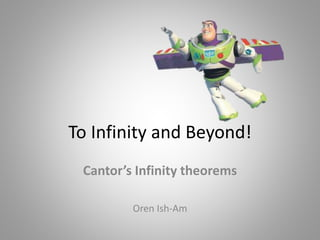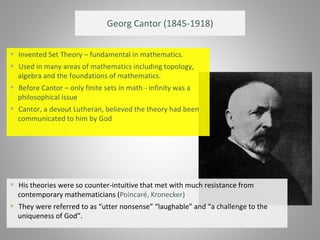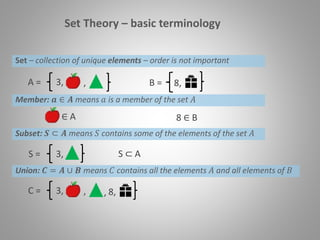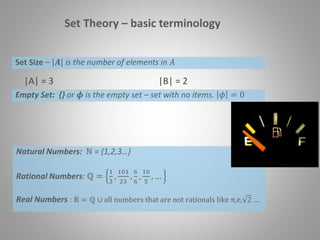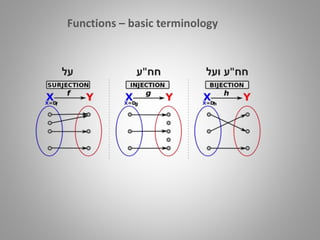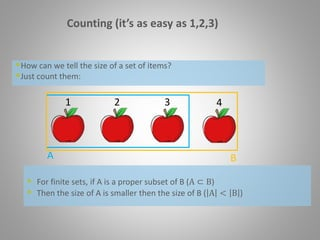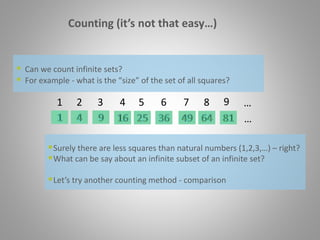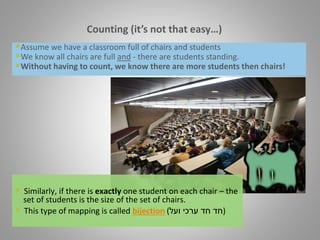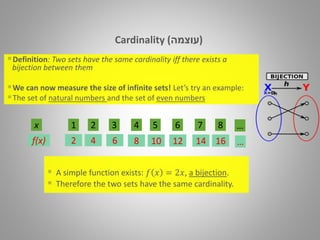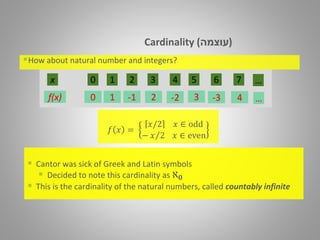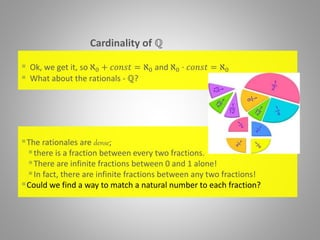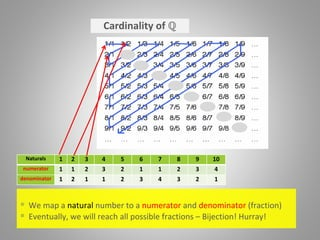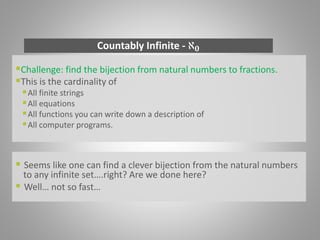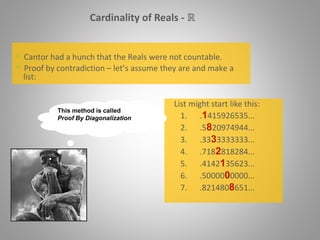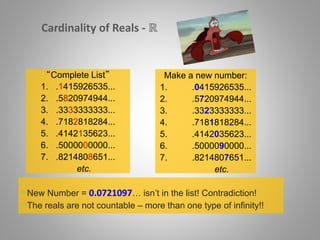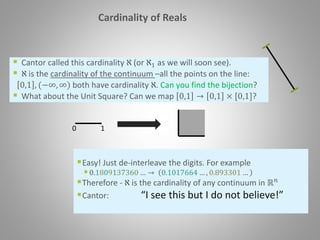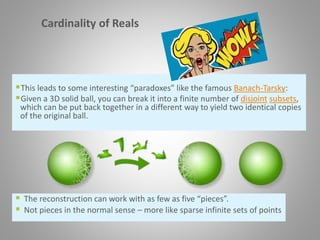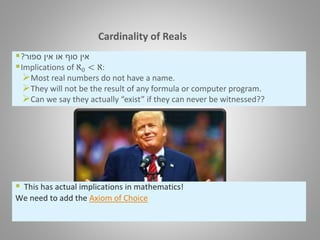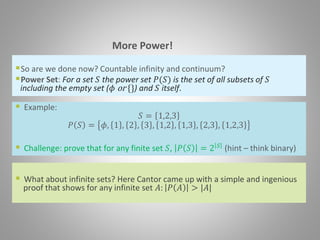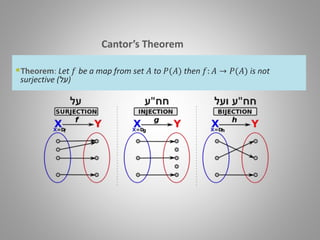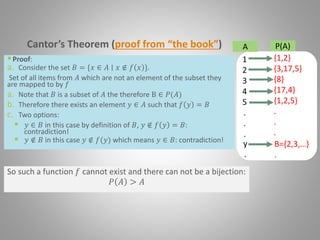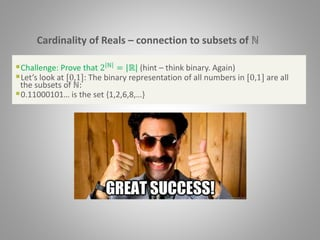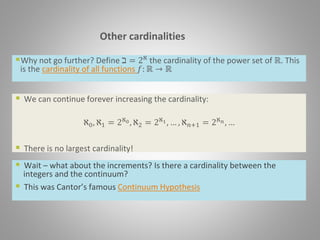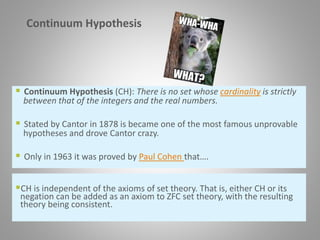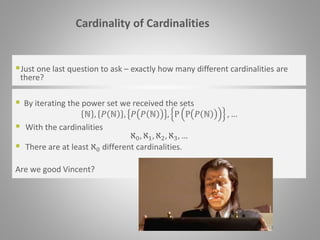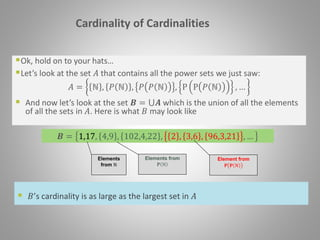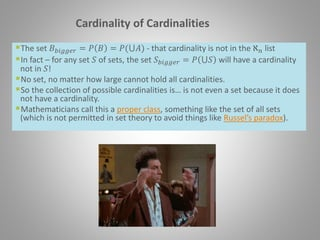1 sur 28

### Cantor Infinity theorems

• 1. To Infinity and Beyond! Cantor’s Infinity theorems Oren Ish-Am
• 2. Georg Cantor (1845-1918)  His theories were so counter-intuitive that met with much resistance from contemporary mathematicians (Poincaré, Kronecker)  They were referred to as “utter nonsense” “laughable” and “a challenge to the uniqueness of God”.  Invented Set Theory – fundamental in mathematics.  Used in many areas of mathematics including topology, algebra and the foundations of mathematics.  Before Cantor – only finite sets in math - infinity was a philosophical issue  Cantor, a devout Lutheran, believed the theory had been communicated to him by God
• 3. Set Theory – basic terminology Set – collection of unique elements – order is not important A = 3, , B = 8, Member: 𝒂 ∈ 𝑨 means 𝑎 is a member of the set 𝐴 ∈ A 8 ∈ B Subset: 𝑺 ⊂ 𝑨 means 𝑆 contains some of the elements of the set 𝐴 S = 3, S ⊂ A Union: 𝑪 = 𝑨 ∪ 𝑩 means 𝐶 contains all the elements 𝐴 and all elements of 𝐵 C = 3, , 8,,
• 4. Set Theory – basic terminology Set Size – |𝑨| is the number of elements in 𝐴 Empty Set: {} or 𝝓 is the empty set – set with no items. 𝜙 = 0 |A| = 3 |B| = 2 Natural Numbers: ℕ = {1,2,3…} Rational Numbers: ℚ = 1 3 , 101 23 , 6 6 , 10 5 , … Real Numbers : ℝ = ℚ ∪ all numbers that are not rationals like π,e, 2 …
• 5. Functions – basic terminology ‫על‬ ‫חח‬"‫ע‬ ‫חח‬"‫ע‬‫ועל‬
• 6. Counting (it’s as easy as 1,2,3) How can we tell the size of a set of items? Just count them: 21 3 4 A B  For finite sets, if A is a proper subset of B (A ⊂ B)  Then the size of A is smaller then the size of B ( A < B )
• 7. Counting (it’s not that easy…) Surely there are less squares than natural numbers (1,2,3,…) – right? What can be say about an infinite subset of an infinite set? Let’s try another counting method - comparison 1 2 3 4 5 6 7 8 9 … …  Can we count infinite sets?  For example - what is the “size” of the set of all squares?
• 8. Assume we have a classroom full of chairs and students We know all chairs are full and - there are students standing. Without having to count, we know there are more students then chairs! Counting (it’s not that easy…)  Similarly, if there is exactly one student on each chair – the set of students is the size of the set of chairs.  This type of mapping is called bijection ( ‫חד‬‫חד‬‫ועל‬ ‫ערכי‬ )
• 9. Cardinality )‫עוצמה‬( Definition: Two sets have the same cardinality iff there exists a bijection between them We can now measure the size of infinite sets! Let’s try an example: The set of natural numbers and the set of even numbers 2 4 6 8 10 12 14 16 …f(x) 1 2 3 4 5 6 7 8 …x  A simple function exists: 𝑓 𝑥 = 2𝑥, a bijection.  Therefore the two sets have the same cardinality.
• 10. Cardinality )‫עוצמה‬( How about natural number and integers? 1 -1 2 -2 3 -3 4 …f(x) 0 1 2 3 4 5 6 7 …x 0 𝑓 𝑥 = 𝑥/2 𝑥 ∈ odd − 𝑥 2 𝑥 ∈ even  Cantor was sick of Greek and Latin symbols  Decided to note this cardinality as ℵ 𝟎  This is the cardinality of the natural numbers, called countably infinite
• 11. Cardinality of ℚ The rationales are dense; there is a fraction between every two fractions. There are infinite fractions between 0 and 1 alone! In fact, there are infinite fractions between any two fractions! Could we find a way to match a natural number to each fraction?  Ok, we get it, so ℵ0 + 𝑐𝑜𝑛𝑠𝑡 = ℵ0 and ℵ0 ⋅ 𝑐𝑜𝑛𝑠𝑡 = ℵ0  What about the rationals - ℚ?
• 12. Cardinality of ℚ Naturals 1 2 3 4 5 6 7 8 9 10 numerator 1 1 2 3 2 1 1 2 3 4 denominator 1 2 1 1 2 3 4 3 2 1  We map a natural number to a numerator and denominator (fraction)  Eventually, we will reach all possible fractions – Bijection! Hurray!
• 13. Countably Infinite - ℵ 𝟎 Challenge: find the bijection from natural numbers to fractions. This is the cardinality of All finite strings All equations All functions you can write down a description of All computer programs.  Seems like one can find a clever bijection from the natural numbers to any infinite set….right? Are we done here?  Well… not so fast…
• 14. Cardinality of Reals - ℝ List might start like this: 1. .1415926535... 2. .5820974944... 3. .3333333333... 4. .7182818284... 5. .4142135623... 6. .5000000000... 7. .8214808651...  Cantor had a hunch that the Reals were not countable.  Proof by contradiction – let’s assume they are and make a list: This method is called Proof By Diagonalization
• 15. Cardinality of Reals - ℝ New Number = 0.0721097… isn’t in the list! Contradiction! The reals are not countable – more than one type of infinity!! “Complete List” 1. .1415926535... 2. .5820974944... 3. .3333333333... 4. .7182818284... 5. .4142135623... 6. .5000000000... 7. .8214808651... etc. Make a new number: 1. .0415926535... 2. .5720974944... 3. .3323333333... 4. .7181818284... 5. .4142035623... 6. .5000090000... 7. .8214807651... etc.
• 16. Cardinality of Reals Easy! Just de-interleave the digits. For example 0.1809137360 … → 0.1017664 … , 0.893301 … Therefore - ℵ is the cardinality of any continuum in ℝ 𝑛 Cantor: “I see this but I do not believe!” 0 1  Cantor called this cardinality ℵ (or ℵ1 as we will soon see).  ℵ is the cardinality of the continuum –all the points on the line: 0,1 , (−∞, ∞) both have cardinality ℵ. Can you find the bijection?  What about the Unit Square? Can we map 0,1 → 0,1 × [0,1]?
• 17. Cardinality of Reals This leads to some interesting “paradoxes” like the famous Banach-Tarsky: Given a 3D solid ball, you can break it into a finite number of disjoint subsets, which can be put back together in a different way to yield two identical copies of the original ball.  The reconstruction can work with as few as five “pieces”.  Not pieces in the normal sense – more like sparse infinite sets of points
• 18. Cardinality of Reals  ‫ספור‬ ‫אין‬ ‫או‬ ‫סוף‬ ‫אין‬? Implications of ℵ0 < ℵ: Most real numbers do not have a name. They will not be the result of any formula or computer program. Can we say they actually “exist” if they can never be witnessed??  This has actual implications in mathematics! We need to add the Axiom of Choice
• 19. More Power! So are we done now? Countable infinity and continuum? Power Set: For a set 𝑆 the power set 𝑃(𝑆) is the set of all subsets of 𝑆 including the empty set (𝜙 or {}) and 𝑆 itself.  Example: 𝑆 = 1,2,3 𝑃 𝑆 = 𝜙, 1 , 2 , 3 , 1,2 , 1,3 , 2,3 , 1,2,3  Challenge: prove that for any finite set 𝑆, 𝑃 𝑆 = 2 𝑆 (hint – think binary)  What about infinite sets? Here Cantor came up with a simple and ingenious proof that shows for any infinite set 𝐴: 𝑃 𝐴 > |𝐴|
• 20. Cantor’s Theorem Theorem: Let 𝑓 be a map from set 𝐴 to 𝑃(𝐴) then 𝑓: 𝐴 → 𝑃(𝐴) is not surjective (‫)על‬ ‫על‬ ‫חח‬"‫ע‬ ‫חח‬"‫ע‬‫ועל‬
• 21. Cantor’s Theorem (proof from “the book”) Proof: a. Consider the set 𝐵 = {𝑥 ∈ 𝐴 ∣ 𝑥 ∉ 𝑓 𝑥 }. Set of all items from 𝐴 which are not an element of the subset they are mapped to by 𝑓 a. Note that 𝐵 is a subset of 𝐴 the therefore B ∈ 𝑃(𝐴) b. Therefore there exists an element 𝑦 ∈ 𝐴 such that 𝑓 𝑦 = 𝐵 c. Two options:  𝑦 ∈ 𝐵 in this case by definition of 𝐵, 𝑦 ∉ 𝑓 𝑦 = 𝐵: contradiction!  𝑦 ∉ 𝐵 in this case 𝑦 ∉ 𝑓(𝑦) which means 𝑦 ∈ 𝐵: contradiction! So such a function 𝑓 cannot exist and there can not be a bijection: 𝑃 𝐴 > 𝐴 1 2 3 4 5 . . . {1,2} {3,17,5} {8} {17,4} {1,2,5} . . . y . B={2,3,…} . A P(A)
• 22. Cardinality of Reals – connection to subsets of ℕ Challenge: Prove that 2 ℕ = |ℝ| (hint – think binary. Again) Let’s look at [0,1]: The binary representation of all numbers in [0,1] are all the subsets of ℕ: 0.11000101… is the set {1,2,6,8,…}
• 23. Other cardinalities Why not go further? Define ℶ = 2ℵ the cardinality of the power set of ℝ. This is the cardinality of all functions 𝑓: ℝ → ℝ  We can continue forever increasing the cardinality: ℵ0, ℵ1 = 2ℵ0, ℵ2 = 2ℵ1, … , ℵ 𝑛+1 = 2ℵ 𝑛, …  There is no largest cardinality!  Wait – what about the increments? Is there a cardinality between the integers and the continuum?  This was Cantor’s famous Continuum Hypothesis
• 24. Continuum Hypothesis CH is independent of the axioms of set theory. That is, either CH or its negation can be added as an axiom to ZFC set theory, with the resulting theory being consistent.  Continuum Hypothesis (CH): There is no set whose cardinality is strictly between that of the integers and the real numbers.  Stated by Cantor in 1878 is became one of the most famous unprovable hypotheses and drove Cantor crazy.  Only in 1963 it was proved by Paul Cohen that….
• 25. Cardinality of Cardinalities Just one last question to ask – exactly how many different cardinalities are there?  By iterating the power set we received the sets ℕ , 𝑃 ℕ , 𝑃 𝑃 ℕ , P P 𝑃 ℕ , …  With the cardinalities ℵ0, ℵ1, ℵ2, ℵ3, …  There are at least ℵ0 different cardinalities. Are we good Vincent?
• 26. 𝑩 = 1,17, 4,9 , 102,4,22 , 2 , 3,6 , 96,3,21 , … Cardinality of Cardinalities Ok, hold on to your hats… Let’s look at the set 𝐴 that contains all the power sets we just saw: 𝐴 = ℕ , 𝑃 ℕ , 𝑃 𝑃 ℕ , P P 𝑃 ℕ , …  And now let’s look at the set 𝑩 = ⋃𝑨 which is the union of all the elements of all the sets in 𝐴. Here is what 𝐵 may look like Elements from ℕ Elements from 𝐏 ℕ Element from 𝐏 𝐏 ℕ  𝐵’s cardinality is as large as the largest set in 𝐴
• 27. Cardinality of Cardinalities The set 𝐵 𝑏𝑖𝑔𝑔𝑒𝑟 = 𝑃 𝐵 = 𝑃(⋃𝐴) - that cardinality is not in the ℵ 𝑛 list In fact – for any set 𝑆 of sets, the set 𝑆 𝑏𝑖𝑔𝑔𝑒𝑟 = 𝑃 ⋃𝑆 will have a cardinality not in 𝑆! No set, no matter how large cannot hold all cardinalities. So the collection of possible cardinalities is… is not even a set because it does not have a cardinality. Mathematicians call this a proper class, something like the set of all sets (which is not permitted in set theory to avoid things like Russel’s paradox).
• 28. Thank You!

### Notes de l'éditeur

1. Give slide with basic definitions – Set, member, Union, Subset, Intersection. And other set notation
2. Add animation to cross off the main diagonal for repetition